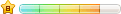返回论坛 充值金币 发帖赚钱 重要说明 举报此贴 打赏高手 关闭本页
 温馨提示:【金牌平坛】地址 是： https://438380.com 或 https://www.438390.com 敬请留意。
 主题 : 106期：【生命的叹息の五不中】走过路过不要错过 阅读7640次 |发帖月入十万不是

# 106期：【生命的叹息の五不中】走过路过不要错过

098期： 【生命的叹息の五不中】<<49.16.08.28.22>> 开40+15+21+10+44+17 = 12
099期： 【生命的叹息の五不中】<<22.01.37.03.13>> 开48+20+45+25+39+15 = 28
100期： 【生命的叹息の五不中】<<23.39.22.04.14>> 开24+05+20+02+27+32 = 12
101期： 【生命的叹息の五不中】<<28.35.04.19.09>> 开25+29+22+20+49+24 = 36
102期： 【生命的叹息の五不中】<<21.10.43.19.17>> 开06+19+04+39+42+32 = 34 错
103期： 【生命的叹息の五不中】<<47.18.09.49.26>> 开12+32+16+04+10+30 = 14
104期： 【生命的叹息の五不中】<<38.48.19.12.36>> 开38+31+27+17+32+30 = 11 错
105期： 【生命的叹息の五不中】<<16.37.13.09.17>> 开41+24+40+34+10+45 = 35
106期： 【生命的叹息の五不中】<<32.28.40.15.34>> 开00
[ 此帖被生命的叹息在2023-09-17 18:48:18重新编辑 ]
<<    1     2    3    4    5   >> [共22页]